• CNN图像分类 千次阅读
2020-12-15 19:51:31

Tensorflow提供的图像分类工具

Tensorflow提供了一个能基于预训练模型进行重训练的脚本：https://github.com/tensorflow/hub/blob/master/examples/image_retraining/retrain.py，只需提供图像即可完成训练，不用写代码，预训练模型可以使用inception v3，或者准确率相对较低但运行更快的mobilenet。

目前这个脚本已经有了针对tensorflow 2.0的更新版本：make_image_classifier.py

通过以下命令即可完成在几分钟内完成训练：

\$ make_image_classifier \

--image_dir my_image dir \

--image_size 224 \

--saved_model_dir my_dir/new_model \

--labels_output_file class_labels.txt \

--tflite_output_file new_mobile_model.tflite

下面讲下参数。

--image_dir，存放训练图像的目录，结构如下：

my image_dir

|-- cat

| |-- a_feline_photo.jpg

| |-- another_cat_pic.jpg

| -- ...

|-- dog

| |-- walking_the_dog.jpeg

| -- ...

-- rabbit

|-- IMG87654321.JPG

|-- my_fluffy_rabbit.JPEG

-- ...

--tfhub_module，指定使用的预训练模型，可以在tfhub上搜索到可以用的各种模型。

--image_size，图像会被缩放以满足模型的输入要求，这个参数应该和预训练模型的吻合。

--saved_model_dir，将模型保存为Tensorflow Savedmodel格式，可以用于Tensorflow Serving服务器。

--tflite_output_file，将模型保存为Tensorflow Lite格式。

我用它训练了一个文字图像识别器，主要有两个类别：文字图像、非文字图像。因为有的文字图像并不是规则的正方形，还需要进行适当的裁剪。

下面是调用生成的Tensorflow Lite模型进行识别的Python脚本。

"""label_image for tflite."""

from __future__ import absolute_import

from __future__ import division

from __future__ import print_function

import cv2

import argparse

import numpy as np

import tensorflow as tf # TF2

def imgData(filename,new_size):

h=image.shape

w=image.shape

centerX=int(w/2)

centerY=int(h/2)

if h/w>1.5:

image=image[int(centerY-w/2):int(centerY+w/2)]

if w/h>1.5:

image=image[:,int(centerX-h/2):int(centerX+h/2)]

data=cv2.resize(image, new_size, interpolation = cv2.INTER_LINEAR)

input_data=data[np.newaxis,:,:,:]

return input_data

with open(filename, 'r') as f:

return [line.strip() for line in f.readlines()]

if __name__ == '__main__':

parser = argparse.ArgumentParser()

'-i',

'--image',

default='/tmp/grace_hopper.bmp',

help='image to be classified')

'-m',

'--model_file',

default='/tmp/mobilenet_v1_1.0_224_quant.tflite',

help='.tflite model to be executed')

'-l',

'--label_file',

default='/tmp/labels.txt',

help='name of file containing labels')

'--input_mean',

default=127.5, type=float,

help='input_mean')

'--input_std',

default=127.5, type=float,

help='input standard deviation')

args = parser.parse_args()

interpreter = tf.lite.Interpreter(model_path=args.model_file)

interpreter.allocate_tensors()

input_details = interpreter.get_input_details()

output_details = interpreter.get_output_details()

# check the type of the input tensor floating_model = input_details['dtype'] == np.float32

# NxHxWxC, H:1, W:2 height = input_details['shape']

width = input_details['shape']

new_size = (height, width)

input_data=imgData(args.image,new_size)

if floating_model:

input_data = (np.float32(input_data) - args.input_mean) / args.input_std

interpreter.set_tensor(input_details['index'], input_data)

interpreter.invoke()

output_data = interpreter.get_tensor(output_details['index'])

results = np.squeeze(output_data)

top_k = results.argsort()[-5:][::-1]

for i in top_k:

if floating_model:

print('{:08.6f}: {}'.format(float(results[i]), labels[i]))

else:

print('{:08.6f}: {}'.format(float(results[i] / 255.0), labels[i]))

用法：

python label_image.py \

--input_mean 0 --input_std 255 \

--model_file new_mobile_model.tflite --label_file class_labels.txt \

--image my_image_dir/cat/a_feline_photo.jpg

更多相关内容
• 有监督学习，图像分类，训练预测主程序，图像为手写数据
• CNN实现对FashionMNIST图像分类 卷积神经网络相对于全连接神经网络的优势： 参数少 -> 权值共享 因为全连接神经网络输入的图片像素较大， 所以参数较多 而卷积神经网络的参数主要在于核上， 而且核的参数可以共享给...
• 主要介绍了Pytorch 使用CNN图像分类的实现，文中通过示例代码介绍的非常详细，对大家的学习或者工作具有一定的参考学习价值，需要的朋友们下面随着小编来一起学习学习吧
• cnn 图像分类PPTcnn 图像分类PPTcnn 图像分类PPTcnn 图像分类PPTcnn 图像分类PPTcnn 图像分类PPTcnn 图像分类PPTcnn 图像分类PPTcnn 图像分类PPTcnn 图像分类PPT
• 迁移学习CNN图像分类模型 - 花朵图片分类-附件资源
• 文章目录TensorFlow2 学习——CNN图像分类1. 导包2. 图像分类 fashion_mnist3. 图像分类 Dogs vs. Cats3.1 原始数据3.2 利用Dataset加载图片3.3 构建CNN模型，并训练 TensorFlow2 学习——CNN图像分类 1. 导包 ...
• 本篇文章了tensorflow训练自己的数据集实现CNN图像分类，小编觉得挺不错的，现在分享给大家，也给大家做个参考。一起跟随小编过来看看吧
• 人工智能深度学习用于图像分类神经网络 matlab平台 方便快捷 好用
• cnn图像分类PyTorch on CIFAR10 CIFAR10上的PyTorch In my previous posts we have gone through 在我以前的帖子中，我们已经看过 Deep Learning — Artificial Neural Network(ANN) 深度学习-人工神经网络(ANN) ...

cnn图像分类

PyTorch on CIFAR10

CIFAR10上的PyTorch

In my previous posts we have gone through

在我以前的帖子中，我们已经看过

Let us try to classify images using Convolution

让我们尝试使用卷积对图像进行分类

# 2D卷积：运算 (2D Convolutions: The operation)

The idea behind convolution is the use of image kernels. A kernel is a small matrix (usually of size 3 by 3) used to apply effect to an image (like sharpening, blurring…).They’re also used in machine learning for ‘feature extraction’, a technique for determining the most important portions of an image.

卷积背后的想法是使用图像内核。 核是用于对图像施加效果(如锐化，模糊等)的小矩阵(通常为3×3)。它们还用于机器学习中的“特征提取”，这是一种确定最重要的技术。图片的一部分。

Common techniques used in CNN : Padding and Striding

CNN中使用的常用技术：填充和跨步

Padding: If you see the animation above, notice that during the sliding process, the edges essentially get “trimmed off”, converting a 5×5 feature matrix to a 3×3 one.

填充 ：如果您看到上面的动画，请注意，在滑动过程中，边缘实际上被“修剪掉了”，将5×5特征矩阵转换为3×3特征矩阵。

This is how padding works, pad the edges with extra, “fake” pixels (usually of value 0, hence the oft-used term “zero padding”). This way, the kernel when sliding can allow the original edge pixels to be at its center, while extending into the fake pixels beyond the edge, producing an output the same size as the input.

这就是填充的工作方式，用额外的“假”像素(通常值为0，因此经常使用的术语“零填充”)填充边缘。 这样，内核在滑动时可以允许原始边缘像素位于其中心，同时扩展到边缘以外的伪像素，从而产生与输入大小相同的输出。

Striding: Often when running a convolution layer, you want an output with a lower size than the input. This is commonplace in convolutional neural networks, where the size of the spatial dimensions are reduced when increasing the number of channels. One way of accomplishing this is by using a pooling layer (eg. taking the average/max of every 2×2 grid to reduce each spatial dimensions in half). Yet another way to do is is to use a stride:

跨步 ：通常在运行卷积层时，您希望输出的大小小于输入的大小。 这在卷积神经网络中很常见，在卷积神经网络中，当增加通道数时，空间尺寸的大小会减小。 实现此目的的一种方法是使用池化层(例如，采用每2×2网格的平均值/最大值将每个空间尺寸减半)。 另一方法是大步前进：

The idea of the stride is to skip some of the slide locations of the kernel. A stride of 1 means to pick slides a pixel apart, so basically every single slide, acting as a standard convolution. A stride of 2 means picking slides 2 pixels apart, skipping every other slide in the process, downsizing by roughly a factor of 2, a stride of 3 means skipping every 2 slides, downsizing roughly by factor 3, and so on.

跨步的想法是跳过内核的某些滑动位置。 跨度为1表示将幻灯片分割为一个像素，因此基本上每个幻灯片都作为标准卷积。 跨度为2表示选择相距2个像素的幻灯片，在此过程中跳过其他每张幻灯片，将尺寸缩小约2倍，跨度为3表示将每2张幻灯片跳过，将尺寸缩小3倍，依此类推。

The Multi Channel RGB Image version

多通道RGB图像版本

We deal with RGB images most of the time.In practicality, most input images have 3 channels, and that number only increases the deeper you go into a network

我们大多数时候都处理RGB图像。实际上，大多数输入图像都有3个通道，并且这个数字只会增加您进入网络的深度

Each filter actually happens to be a collection of kernels, with there being one kernel for every single input channel to the layer, and each kernel being unique.Hence for a RGB image we have 3 input channels and 3 kernels which together makes a filter.

每个过滤器实际上都是一个内核集合 该层的每个输入通道都有一个内核，并且每个内核都是唯一的。因此对于RGB图像，我们有3个输入通道和3个内核一起构成一个滤镜。

Each of the kernels of the filter “slides” over their respective input channels, producing a processed version of each. Some kernels may have stronger weights than others, to give more emphasis to certain input channels than others.

过滤器的每个内核在其各自的输入通道上“滑动”，从而生成每个的处理后版本。 一些内核可能比其他内核具有更强的权重，从而比其他内核更强调某些输入通道。

Each of the per-channel processed versions are then summed together to form one channel. The kernels of a filter each produce one version of each channel, and the filter as a whole produces one overall output channel.

然后将每个按通道处理的版本相加在一起以形成一个通道。 过滤器的内核各自为每个通道生成一个版本，而过滤器作为一个整体将生成一个整体输出通道。

Finally, then there’s the bias term. The way the bias term works here is that each output filter has one bias term. The bias gets added to the output channel so far to produce the final output channel.

最后，还有偏差项。 偏置项在这里的工作方式是每个输出滤波器都有一个偏置项。 到目前为止，偏置已添加到输出通道，以产生最终的输出通道。

To dive more into CNN please go through this wonderful article,Intuitively understanding Convolutions for Deep Learning by Irhum Shafkat.

要深入研究CNN，请阅读这篇精彩的文章，Irhum Shafkat的《 直观理解深度学习卷积》

Lets get into coding of CNN with PyTorch.

让我们用PyTorch编码CNN。

Step 1 : Import necessary libraries & Explore the data set

第1步：导入必要的库并浏览数据集

We are importing the necessary libraries pandas , numpy , matplotlib ,torch ,torchvision. With basic EDA we could infer that CIFAR-10 data set contains 10 classes of image, with training data set size of 50000 images , test data set size of 10000.Each image is of [3 x 32 x 32 ]. Which represents 3 channels RGB,32 x 32 pixel size.

我们正在导入必要的库pandas，numpy，matplotlib，torch，torchvision。 使用基本的EDA，我们可以推断出CIFAR-10数据集包含10类图像，训练数据集大小为50000图像，测试数据集大小为10000。每个图像为[3 x 32 x 32]。 代表3通道RGB，32 x 32像素大小。

Step 2 : Prepare data for training

步骤2：准备训练资料

We using training set , validation set , Test set. Why we need them ?

我们使用训练集，验证集，测试集。 为什么我们需要它们？

Training set : used to train our model,computing loss & adjust weights Validation set : To evaluate the model with hyper parameters & pick the best model during training. we are using 10% of training data as validation set Test data set : Used to compare different models & report the final accuracy.

训练集：用于训练我们的模型，计算损失和调整权重验证集：使用超参数评估模型并在训练过程中选择最佳模型。 我们使用10％的训练数据作为验证集测试数据集：用于比较不同的模型并报告最终准确性。

These 2 steps are exactly the same which we have done in our previous logistic regression model.

这两个步骤与我们先前的逻辑回归模型中所做的完全相同。

Step 3: Define CNN model

步骤3：定义CNN模型

The Conv2d layer transforms a 3-channel image to a 16-channel feature map, and the MaxPool2d layer halves the height and width. The feature map gets smaller as we add more layers, until we are finally left with a small feature map, which can be flattened into a vector. We can then add some fully connected layers at the end to get vector of size 10 for each image.

Conv2d图层将3通道图像转换为16通道特征图，而MaxPool2d图层将高度和宽度减半。 随着我们添加更多图层，特征图会变小，直到最终剩下一个小的特征图，可以将其展平为矢量。 然后，我们可以在最后添加一些完全连接的层，以获取每个图像的大小为10的向量。

class Cifar10CnnModel(ImageClassificationBase):    def __init__(self):        super().__init__()        self.network = nn.Sequential(            nn.Conv2d(3, 32, kernel_size=3, padding=1),            nn.ReLU(),            nn.Conv2d(32, 64, kernel_size=3, stride=1, padding=1),            nn.ReLU(),            nn.MaxPool2d(2, 2), # output: 64 x 16 x 16            nn.Conv2d(64, 128, kernel_size=3, stride=1, padding=1),            nn.ReLU(),            nn.Conv2d(128, 128, kernel_size=3, stride=1, padding=1),            nn.ReLU(),            nn.MaxPool2d(2, 2), # output: 128 x 8 x 8            nn.Conv2d(128, 256, kernel_size=3, stride=1, padding=1),            nn.ReLU(),            nn.Conv2d(256, 256, kernel_size=3, stride=1, padding=1),            nn.ReLU(),            nn.MaxPool2d(2, 2), # output: 256 x 4 x 4            nn.Flatten(),             nn.Linear(256*4*4, 1024),            nn.ReLU(),            nn.Linear(1024, 512),            nn.ReLU(),            nn.Linear(512, 10))    def forward(self, xb):        return self.network(xb)

we’d like these outputs to represent probabilities, but for that the elements of each output row must lie between 0 to 1 and add up to 1, which is clearly not the case here.To convert the output rows into probabilities, we use the softmax function.The 10 outputs for each image can be interpreted as probabilities for the 10 target classes (after applying softmax), and the class with the highest probability is chosen as the label predicted by the model for the input image.

我们希望这些输出代表概率，但为此，每个输出行的元素必须位于0到1之间并加1，这显然不是这种情况。要将输出行转换为概率，我们使用softmax函数。每个图像的10个输出可以解释为10个目标类别的概率(应用softmax之后)，并且选择概率最高的类别作为模型为输入图像预测的标签。

Step 4 : Training on a GPU if available

步骤4：在GPU上进行训练(如果有)

To seamlessly use a GPU, if one is available, we define a couple of helper functions (get_default_device & to_device) and a helper class DeviceDataLoader to move our model & data to the GPU as required.Same as used in previous posts.

def get_default_device():    """Pick GPU if available, else CPU"""    if torch.cuda.is_available():        return torch.device('cuda')    else:        return torch.device('cpu')def to_device(data, device):    """Move tensor(s) to chosen device"""    if isinstance(data, (list,tuple)):        return [to_device(x, device) for x in data]    return data.to(device, non_blocking=True)class DeviceDataLoader():    """Wrap a dataloader to move data to a device"""    def __init__(self, dl, device):        self.dl = dl        self.device = device    def __iter__(self):        """Yield a batch of data after moving it to device"""        for b in self.dl:             yield to_device(b, self.device)    def __len__(self):        """Number of batches"""        return len(self.dl)

Defining DeviceDataLoader for automatically transferring batches of data to the GPU (if available), and use to_device to move our model to the GPU (if available).

train_dl = DeviceDataLoader(train_dl, device)val_dl = DeviceDataLoader(val_dl, device)to_device(model, device);

Step 5: Training model

步骤5：训练模型

Now define an evaluate function, which will perform the validation phase, and a fit function which will perform the entire training process.

现在定义一个评估函数，它将执行验证阶段，以及一个拟合函数，它将执行整个培训过程。

@torch.no_grad()def evaluate(model, val_loader):    model.eval()    outputs = [model.validation_step(batch) for batch in val_loader]    return model.validation_epoch_end(outputs)def fit(epochs, lr, model, train_loader, val_loader, opt_func=torch.optim.SGD):    history = []    optimizer = opt_func(model.parameters(), lr)    for epoch in range(epochs):# Training Phase         model.train()        train_losses = []        for batch in train_loader:            loss = model.training_step(batch)            train_losses.append(loss)            loss.backward()            optimizer.step()            optimizer.zero_grad()# Validation phase        result = evaluate(model, val_loader)        result['train_loss'] = torch.stack(train_losses).mean().item()        model.epoch_end(epoch, result)        history.append(result)    return history

Before training let us instantiate the model and see how it performs on the validation set with the initial set of parameters.

在训练之前，让我们实例化模型，并查看其如何在具有初始参数集的验证集中执行。

The initial accuracy is around 10%, which is what one might expect from a randomly initialized model.

初始精度约为10％，这可能是随机初始化模型所期望的。

We are using hyperparmeters , learning rate =0.001 usually set between 0.0001 and 0.1,number of epochs = 10,optimization function used is adam,batch size 128 is used. These values can be changed and experimented to achieve higher accuracy in shorter time.

Evaluating the accuracy vs number of epochs.Our model reaches an accuracy of around 75%, and by looking at the graph, it seems unlikely that the model will achieve an accuracy higher than 80% even after training for a long time. This suggests that we might need to use a more powerful model to capture the relationship between the images and the labels more accurately. This can be done by adding more convolutional layers to our model, or incrasing the no. of channels in each convolutional layer, or by using regularization techniques.

评估精度与历时数的比较我们的模型达到了约75％的精度，通过查看图表，即使经过长时间的训练，该模型也不太可能达到80％以上的精度。 这表明我们可能需要使用功能更强大的模型来更准确地捕获图像和标签之间的关系。 这可以通过在模型中添加更多卷积层或增加No来完成。 每个卷积层中的通道数，或使用正则化技术。

Plotting losses vs number of epochs. Initially, both the training and validation losses seem to decrease over time. However, if you train the model for long enough, you will notice that the training loss continues to decrease, while the validation loss stops decreasing, and even starts to increase after a certain point!

绘制损失与历时数的关系。 最初，训练和验证损失似乎都随着时间而减少。 但是，如果对模型进行足够长时间的训练，您会注意到训练损失继续减少，而验证损失则停止减少，甚至在某个点之后开始增加！

This phenomenon is called overfitting, and it is the no. 1 why many machine learning models give rather terrible results on real-world data. It happens because the model, in an attempt to minimize the loss, starts to learn patters are are unique to the training data, sometimes even memorizing specific training examples.

这种现象称为过拟合 ，但不是。 1为什么许多机器学习模型会在真实数据上给出相当糟糕的结果。 之所以发生这种情况，是因为该模型试图将损失降到最低，从而开始学习训练数据所特有的模式，有时甚至记住特定的训练示例。

Following are some common strategies for avoiding overfitting:

以下是一些避免过度拟合的常用策略：

• Gathering and generating more training data, or adding noise to it

收集并生成更多训练数据，或在其中添加噪音
• Using regularization techniques like batch normalization & dropout

使用正则化技术(例如批量归一化和辍学)
• Early stopping of model’s training, when validation loss starts to increase

当验证损失开始增加时，提早停止模型训练

I will explain in the next post about reaching an accuracy of more than 90% with minor changes in our model.

我将在下一篇文章中解释，通过对模型进行微小的更改，可以达到90％以上的准确性。

步骤6：保存和加载模型

Since we’ve trained our model for a long time and achieved a reasonable accuracy, it would be a good idea to save the weights of the model to disk, so that we can reuse the model later and avoid retraining from scratch.

由于我们已经对模型进行了很长时间的训练并获得了合理的精度，因此将模型的权重保存到磁盘上是一个好主意，这样我们以后就可以重用模型并避免从头开始进行训练。

torch.save(model.state_dict(), 'cifar10-cnn.pth')model2 = to_device(Cifar10CnnModel(), device)model2.load_state_dict(torch.load('cifar10-cnn.pth'))evaluate(model2, test_loader)

Please look into the entire code on notebook Github,Stay connected with me on Linked in.

请仔细阅读笔记本Github上的全部代码，保持联系 已连结

Credits & references :

学分和参考：

cnn图像分类

展开全文计算机视觉 人工智能 python opencv 机器学习
• CNN实现图像分类与识别，包含详细的代码与注释，可以实现人脸识别，手写字符识别等。在较小训练样本条件下CNN获得较高的识别精度。
• cnn-tlvqm-master_神经网络_cnn图像分类_图像分类_CNN_matlabcnn图像.zip
• 基于Pytorch的CNN图像分类器，并利用OpenCV进行数据预处理 CNN（卷积神经网络）在图像处理上有很好的表现，AlphaGO使用也是也是CNN，但是AlphaGO去掉pooling（池化）层。 我以简单的二分类器为例，学习应用CNN进行...

# 数据预处理：

#导入库
import os
import numpy as np
import cv2

# 定义读取图像的函数
# 使用OpenCV库中的.resize()函数修改图像尺寸，为了后面训练神经网络时得到更快的速度
img = cv2.resize(img,(128,128),0)
return img

# 定义函数，将修改后的图像保存
def save(path, img):
# 使用OpenCV库中的.shape()方法获取图像的高和宽，.shape()
rows, cols = img.shape[:2]
# 将图像以一度为步长，旋转360次
for i in range(0,359):
# 使用OpenCV库中的.getRotationMatrix2D()和.warpAffine()方法将图像旋转，以处理数据
# 第一个参数是旋转中心，第二个参数是旋转角度，第三个参数是缩放比例
M = cv2.getRotationMatrix2D((cols/2, rows/2), i, 1)
img1 = cv2.warpAffine(img, M, (cols, rows))
i = str(i)
img_name = i+".jpg"
# 存储图像
cv2.imwrite(os.path.join(path, img_name),img1)

path = '/Users/jason_zhang/Desktop/'

for j in range(1,3):
j = str(j)
no_img = j + '.jpg'
img_path = os.path.join(path, no_img)
new_img_path = os.path.join(path, j)
save(new_img_path ,img)
print("Saving done")


输出结果：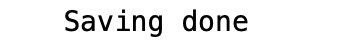# 此时，数据已经处理完，并存出在相应文件夹中。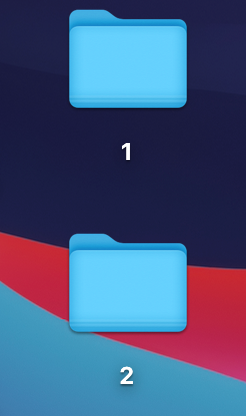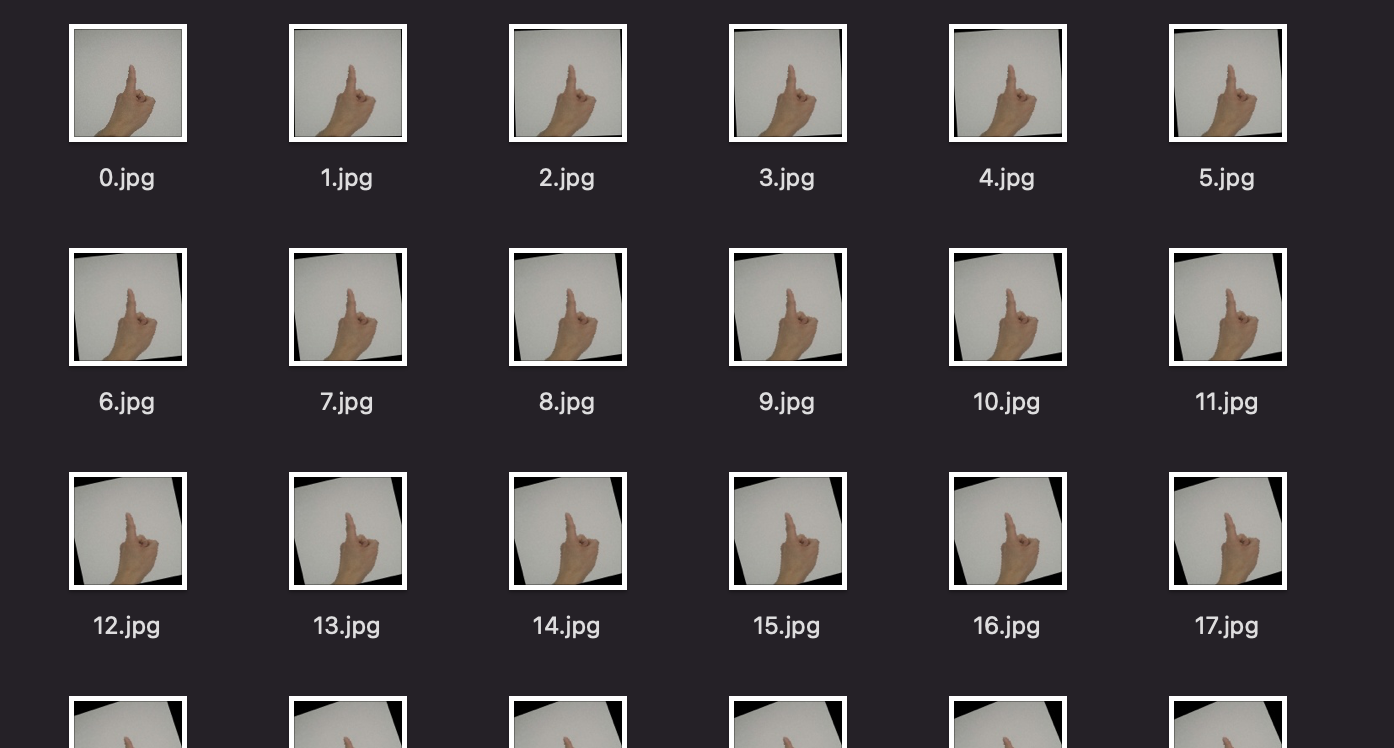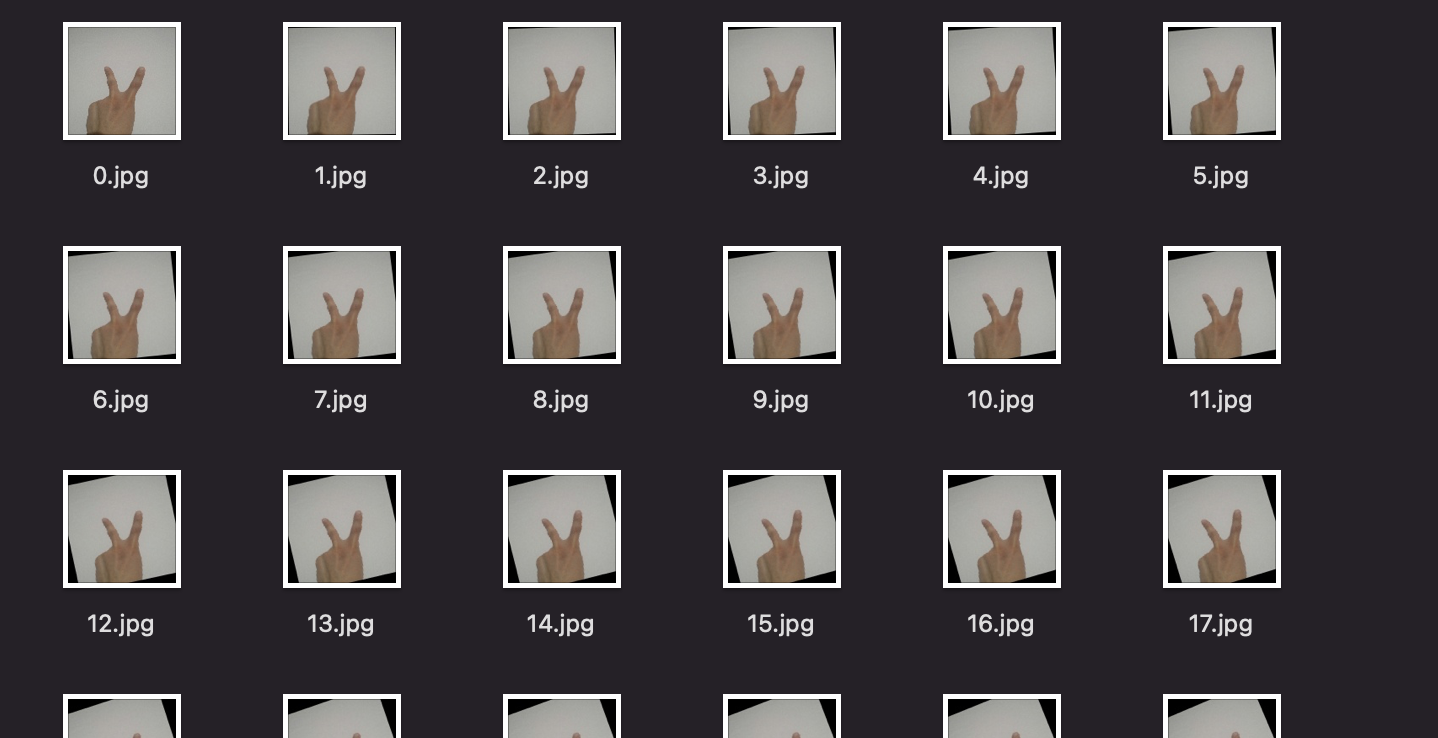# 导入数据：

# 导入库
import os
import numpy as np
import cv2
import torch
import torch.nn as nn
import torchvision.transforms as transforms
import pandas as pd

# 此处的n用于判定lables
n = 1
# 定义读取图像的函数
# 使用sorted()函数，将image的父目录下的图像名称罗列出来，并存储为列表
image_dir = sorted(os.listdir(path))
# 创建features和lables，features为matrices
x = np.zeros((len(image_dir), 128, 128, 3), dtype=np.uint8)
y = np.zeros((len(image_dir)), dtype=np.uint8)
# 使用wnumerate()函数枚举出文件，并为之标号
for i, file in enumerate(image_dir):
x[i, :, :] = cv2.resize(img,(128, 128))
if n == 1:
y[i] = 1
elif n ==2:
y[i] = 2
return x,y

img_dir = '/Users/jason_zhang/Desktop'
# 获取images和lables
# train_是image的matrices
# 使用os库的.path.jion()方法，将image的路径和image的名称连接在一起
# 此处n用于判定lables
n = 2

print("Size of data = {}".format(len(train_x_1)))
print("Size of data = {}".format(len(train_x_2)))


### 输出结果：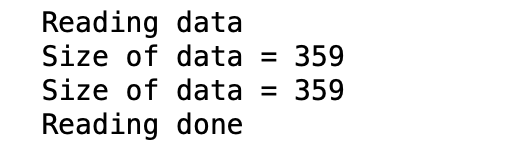# 将两组matrices, lebles分别连接在一起
train_x = np.concatenate([train_x_1, train_x_2], axis = 0)
train_y = np.concatenate([train_y_1, train_y_2], axis = 0)
print("Size of data = {}".format(len(train_x)))


### 输出结果：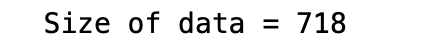# 创建数据集：

# training 时做 data augmentation
train_transform = transforms.Compose([
transforms.ToPILImage(),
transforms.RandomHorizontalFlip(), #随即将图片水平翻转
transforms.RandomRotation(15), #随机旋转图片
transforms.ToTensor(), #將matrices转成 Tensor，並把数值normalize到[0,1](data normalization)
])

# 定义ImgDataset()加载数据
class ImgDataset(Dataset):
def __init__(self, x, y=None, transform=None):
self.x = x
self.y = y
self.transform = transform
def __len__(self):
return len(self.x)
def __getitem__(self, index): # 根据index返回一行数据
X = self.x[index]
if self.transform is not None:
X = self.transform(X)
Y = self.y[index]
return X, Y

# 批量再入数据集
batch_size = 32
train_set = ImgDataset(train_x, train_y, train_transform)


# 定义Classifier分类器

class Classifier(nn.Module):
def __init__(self):
super(Classifier, self).__init__()
# torch.nn.Conv2d(in_channels, out_channels, kernel_size, stride, padding)
# input 维度 [3, 128, 128]
self.cnn = nn.Sequential(
nn.Conv2d(3, 64, 3, 1, 1),  # [64, 128, 128]
nn.BatchNorm2d(64),
nn.ReLU(),
nn.MaxPool2d(2, 2, 0),      # [64, 64, 64]

nn.Conv2d(64, 128, 3, 1, 1), # [128, 64, 64]
nn.BatchNorm2d(128),
nn.ReLU(),
nn.MaxPool2d(2, 2, 0),      # [128, 32, 32]

nn.Conv2d(128, 256, 3, 1, 1), # [256, 32, 32]
nn.BatchNorm2d(256),
nn.ReLU(),
nn.MaxPool2d(2, 2, 0),      # [256, 16, 16]

nn.Conv2d(256, 512, 3, 1, 1), # [512, 16, 16]
nn.BatchNorm2d(512),
nn.ReLU(),
nn.MaxPool2d(2, 2, 0),       # [512, 8, 8]

nn.Conv2d(512, 512, 3, 1, 1), # [512, 8, 8]
nn.BatchNorm2d(512),
nn.ReLU(),
nn.MaxPool2d(2, 2, 0),       # [512, 4, 4]
)
# 定义全连接层
self.fc = nn.Sequential(
nn.Linear(512*4*4, 1024),
nn.ReLU(),
nn.Linear(1024, 512),
nn.ReLU(),
nn.Linear(512, 3)
)
# 定义forward
def forward(self, x):
out = self.cnn(x)
out = out.view(out.size(), -1)
return self.fc(out)


# 训练CNN：

model = Classifier().cuda() # 传送模型到gpu
loss = nn.CrossEntropyLoss() # 因为是 classification task，所以 loss 使用 CrossEntropyLoss
num_epoch = 10

for epoch in range(num_epoch):
train_acc = 0.0
train_loss = 0.0

model.train()
train_pred = model(data.cuda())
data = data.type(torch.LongTensor) # 将lables转换成LongTensor
batch_loss = loss(train_pred, data.cuda())
batch_loss.backward() # backward() 计算梯度
optimizer.step() # 更新参数

# 计算accuracy
train_acc += np.sum(np.argmax(train_pred.cpu().data.numpy(), axis=1) == data.numpy())
train_loss += batch_loss.item()

print('''[%03d/%03d] Train Acc: %3.6f Loss: %3.6f ''' %  (epoch + 1, num_epoch,train_acc/train_set.__len__(), train_loss/train_set.__len__()))


### 输出结果：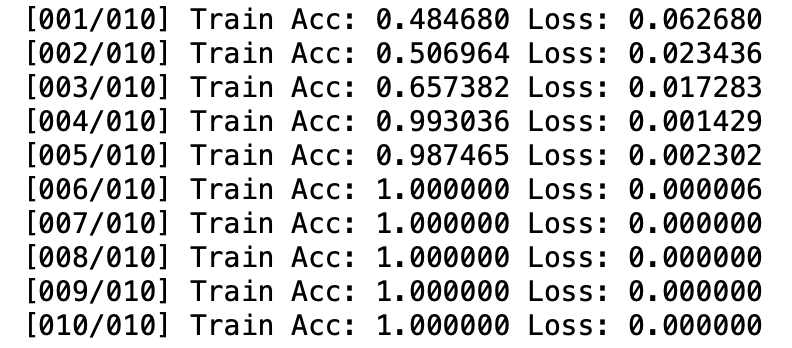# 没有使用validate set 和 test set，是因为只想学习CNN的搭建和训练，以及数据的批量加载，和OpenCV进行图像处理

展开全文神经网络 深度学习 python opencv pytorch
• cnn-tlvqm-master_神经网络_cnn图像分类_图像分类_CNN_matlabcnn图像_源码.zip
• 卷积神经网络图像识别，matlab。包含了卷积神经网络matlab必备的代码部分，可直接运行，无需改变，
• 利用深度学习模型CNN图像进行分类与识别，例如人脸识别，手写字符识别等。
• 用progressive resizing提升CNN图像分类器性能，Boost your CNN image classifier performance with progressive resizing in Keras，by Aleksey Bilogur。
• 卷积神经网络，可以很好的实现文本分类或者图像识别
• 本文将大致介绍经典的卷积神经网络。... 卷积神经网络概述： 相比于传统特征提取方法，卷积不需要人工进行特征提取。...CNN的优点：（1）局部连接。每个神经元只和前一层部分神经元连接，这可以加快网络收敛和减...

## 卷积神经网络概述：

相比于传统特征提取方法，卷积不需要人工进行特征提取。受启发于生物神经元，激活函数用于仿真，当生物电信号超过了某一阈值，就被传递给下一个神经元；损失函数用于指导网络学习到被期望学习的东西。

CNN的优点：（1）局部连接。每个神经元只和前一层部分神经元连接，这可以加快网络收敛和减少参数。这个优点是和全连接网络比较的，全连接层一般会拥有大量的参数，这会使模型过于庞大、优化速度慢、计算量大、过拟合等。（2）权重共享。一组连接可以共享相同的权重。实际上卷积也存在着权重共享，比如使用一个卷积核对前一层的n个通道进行卷积计算，这相当于是通道共享和图共享了卷积。（3）下采样减少维度。池化层通过提取某个区域内最重要的信息（最大值或平均值）来减少数据量。

CNN的主要结构：

（1）输入层。CNN的输入一般是二维向量，可以有高度，比如，RGB图像。
（2）卷积层。卷积层是CNN的核心，层的参数由一组可学习的滤波器（filter）或内核（kernels）组成，它们具有小的感受野，延伸到输入容积的整个深度。卷积层的作用是对输入层进行卷积，提取更高层次的特征。
（3）池化层。池化层（又称为下采样），它的作用是减小数据处理量同时保留有用信息，池化层的作用可以描述为模糊图像，丢掉了一些不是那么重要的特征。池化层一般包括均值池化、最大池化、高斯池化、可训练池化等。
（4）激活层。激活层主要是把卷积层输出结果做非线性映射，常用的激励函数有ReLU、sigmoid、tanh、LeakyReLU等。CNN采用的激励函数一般为ReLU(The Rectified Linear Unit，修正线性单元)，它的特点是收敛快，求梯度简单，但较脆弱。（5）（5）连接层。全连接层是一个常规的神经网络，它的作用是对经过多次卷积层和多次池化层所得出来的高级特征进行全连接（全连接就是常规神经网络的性质），算出最后的预测值。
（6）输出层。输出层输出对结果的预测值，一般会加一个softmax层。

## 经典的CNN模型：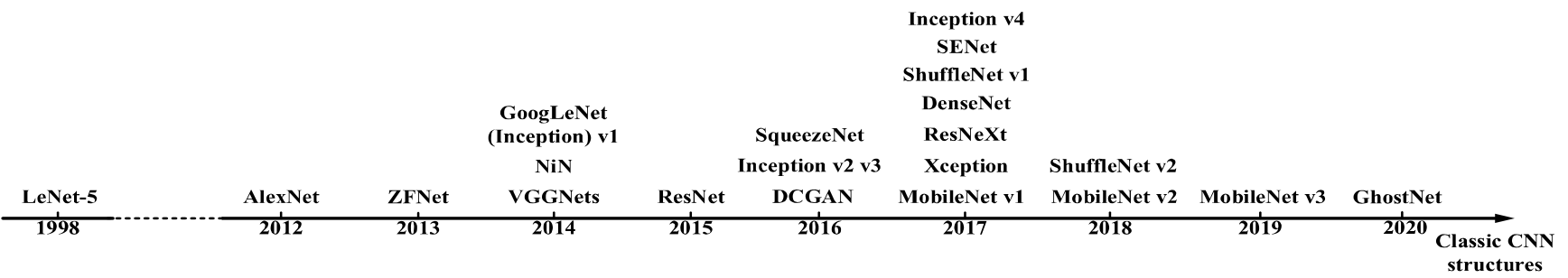### 1. LeNet-5：

被用于手写字符识别。共有7层，2个卷积层，2个池化层，3个全连接层。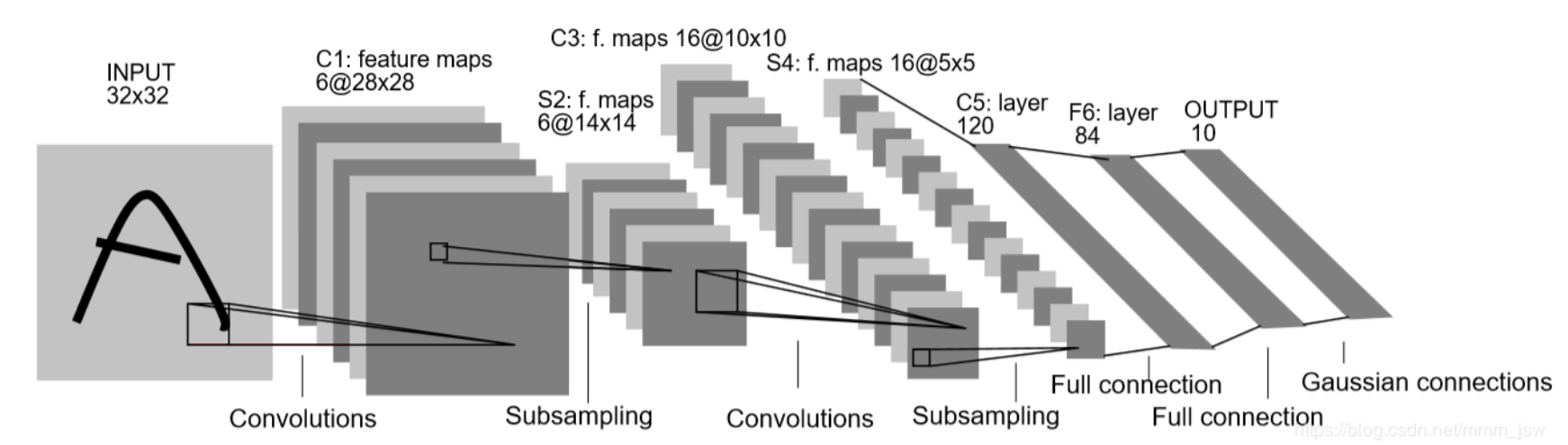LeNet5网络虽然很小，但是包含了深度学习的基本模块：卷积层、池化层、全连接层。LeNet5共有七层，不包含输入，每层都包含可训练参数，每个层有多个Feature Map，每个Feature Map通过一种卷积滤波器提取输入的一种特征，然后每Feature Map有多个神经元。
输入： 32∗32
32∗32的手写字体图片，这些手写字体包含0-9数字，也就是相当于10个类别的图片。
输出： 分类结果，0-9之间的一个数（softmax）

### 2. AlexNet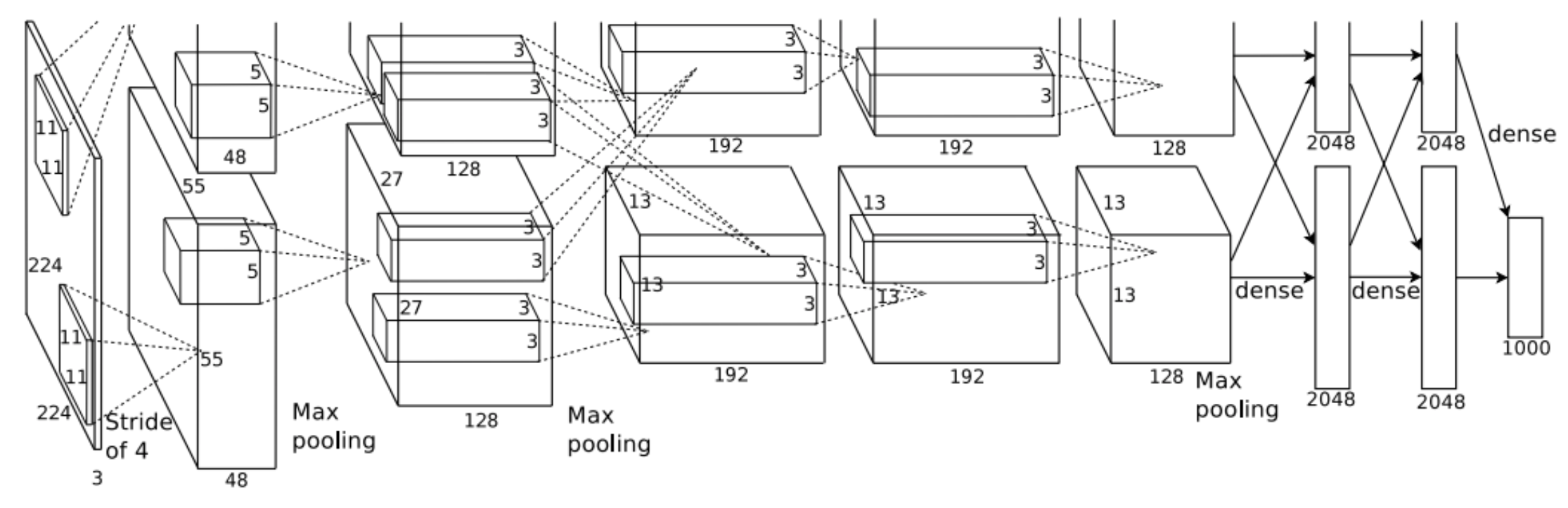AlexNet获得了2012年ImageNet竞赛的冠军。共有8层，包括5个卷积层和3个全连接层。主要创新：

（1）使用ReLU作为激活函数。ReLU及其常见变体

（2）使用了Dropout。

（3）用最大池化替代了平均池化。

（4）使用LRN做normalization，提高模型泛化性。

（5）使用双GPU训练组卷积。

（6）数据增广。

### 3. VGG

Vgg包含了一系列的网络。作者证明了增加网络深度可以提高最终性能。主要创新：

（1）移除了LRN层

（2）使用3x3卷积核替代了5x5卷积。因为两个3x3卷积可以达到和一个5x5相等的感知域，然而参数量更少。

解决的问题：

（1）参数太多，如果训练数据集有限，很容易产生过拟合；

（2）网络越大、参数越多，计算复杂度越大，难以应用；

（3）网络越深，容易出现梯度弥散问题（梯度越往后穿越容易消失），难以优化模型。

解决这些问题的方法当然就是在增加网络深度和宽度的同时减少参数，为了减少参数，自然就想到将全连接变成稀疏连接。但是在实现上，全连接变成稀疏连接后实际计算量并不会有质的提升，因为大部分硬件是针对密集矩阵计算优化的，稀疏矩阵虽然数据量少，但是计算所消耗的时间却很难减少。

创新：

（2）网络最后采用了average pooling（平均池化）来代替全连接层，该想法来自NIN（Network in Network），事实证明这样可以将准确率提高0.6%。但是，实际在最后还是加了一个全连接层，主要是为了方便对输出进行灵活调整；
（3）虽然移除了全连接，但是网络中依然使用了Dropout ;
（4）为了避免梯度消失，网络额外增加了2个辅助的softmax用于向前传导梯度（辅助分类器）。辅助分类器是将中间某一层的输出用作分类，并按一个较小的权重（0.3）加到最终分类结果中，这样相当于做了模型融合，同时给网络增加了反向传播的梯度信号，也提供了额外的正则化，对于整个网络的训练很有裨益。而在实际测试的时候，这两个额外的softmax会被去掉。

### 5.ResNet

深层的网络一般能比浅层网络性能更好。但是网络太深会带来梯度消失和梯度爆炸的问题。VGG网络达到19层后再增加层数就开始导致分类性能的下降。

ResNets要解决的是深度神经网络的“退化”问题。对浅层网络逐渐叠加layers，模型在训练集和测试集上的性能会变好，因为模型复杂度更高了，表达能力更强了，可以对潜在的映射关系拟合得更好。而“退化”指的是，给网络叠加更多的层后，性能却快速下降的情况。有两种解决思路，一种是调整求解方法，比如更好的初始化、更好的梯度下降算法等；另一种是调整模型结构，让模型更易于优化——改变模型结构实际上是改变了error surface的形态。

ResNet设计网络为H(x) = F(x) + x，即直接把恒等映射作为网络的一部分。就可以把问题转化为学习一个残差函数F(x) = H(x) - x.只要F(x)=0，就构成了一个恒等映射H(x) = x。 而且，拟合残差至少比拟合恒等映射容易得多。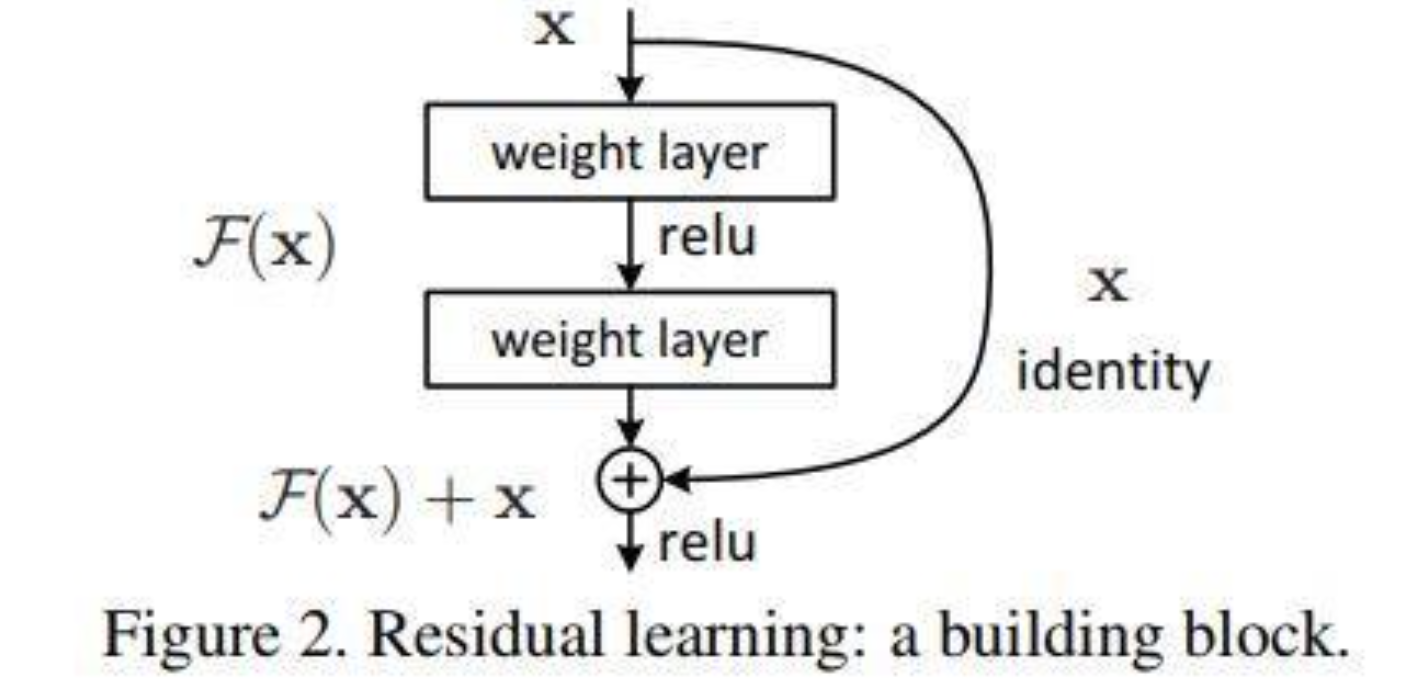未完

参考：

20200409_A Survey of Convolutional Neural Network.

https://blog.csdn.net/mmm_jsw/article/details/88185491

https://my.oschina.net/u/876354/blog/1637819

https://www.cnblogs.com/shine-lee/p/12363488.html

展开全文• 经典网络结构，图像分类，目标检测论文综述、介绍等等。
• matlab的程序cnn编写，对图片进行分类并预测，最后完成搜索功能， 输入图片路径给出所有同类的图片结果
• 最近基于VGG-16缩进了网络做了一个CNN模型用于处理图像分类，实际项目，训练对象是448×32的长条试纸图片。 目录 项目源码百度云 tensorboard可视化展示 源代码 项目源码百度云 项目源码百度云链接：...实际项目
• CNN实现图片分类，用tensorflow python实现，目前是三类，可以增加类别。...